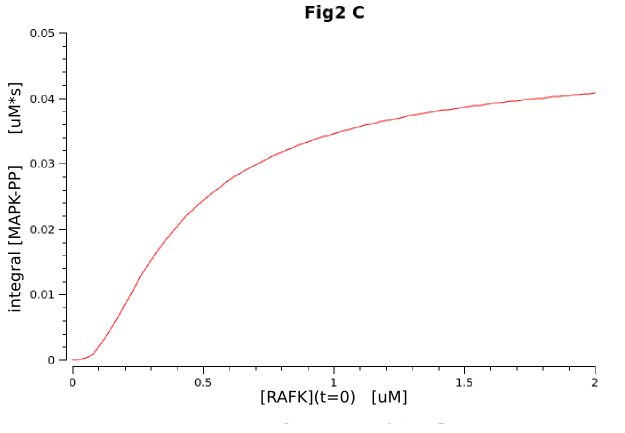## Levchenko2000_MAPK_noScaffoldModel Identifier
BIOMD0000000011
Short description

# MAPK cascade in solution (no scaffold)

Description
This model describes a basic 3- stage Mitogen Activated Protein Kinase (MAPK) cascade in solution. This cascade is typically expressed as RAF= =>MEK==>MAPK (alternative forms are K3==>K2==> K1 and KKK==>KK==>K) . The input signal is RAFK (RAF Kinase) and the output signal is MAPKpp ( doubly phosphorylated form of MAPK) . RAFK phosphorylates RAF once to RAFp. RAFp, the phosphorylated form of RAF induces two phoshporylations of MEK, to MEKp and MEKpp. MEKpp, the doubly phosphorylated form of MEK, induces two phosphorylations of MAPK to MAPKp and MAPKpp.
Rate constant       Reaction
a10 = 5. MAPKPH + MAPKpp -> MAPKppMAPKPH
a1 = 1. RAF + RAFK -> RAFRAFK
a2 = 0.5 RAFp + RAFPH -> RAFpRAFPH
a3 = 3.3 MEK + RAFp -> MEKRAFp
a4 = 10. MEKp + MEKPH -> MEKpMEKPH
a5 = 3.3 MEKp + RAFp -> MEKpRAFp
a6 = 10. MEKPH + MEKpp -> MEKppMEKPH
a7 = 20. MAPK + MEKpp -> MAPKMEKpp
a8 = 5. MAPKp + MAPKPH -> MAPKpMAPKPH
a9 = 20. MAPKp + MEKpp -> MAPKpMEKpp
d10 = 0.4 MAPKppMAPKPH -> MAPKPH + MAPKpp
d1 = 0.4 RAFRAFK -> RAF + RAFK
d2 = 0.5 RAFpRAFPH -> RAFp + RAFPH
d3 = 0.42 MEKRAFp -> MEK + RAFp
d4 = 0.8 MEKpMEKPH -> MEKp + MEKPH
d5 = 0.4 MEKpRAFp -> MEKp + RAFp
d6 = 0.8 MEKppMEKPH -> MEKPH + MEKpp
d7 = 0.6 MAPKMEKpp -> MAPK + MEKpp
d8 = 0.4 MAPKpMAPKPH -> MAPKp + MAPKPH
d9 = 0.6 MAPKpMEKpp -> MAPKp + MEKpp
k10 = 0.1 MAPKppMAPKPH -> MAPKp + MAPKPH
k1 = 0.1 RAFRAFK -> RAFK + RAFp
k2 = 0.1 RAFpRAFPH -> RAF + RAFPH
k3 = 0.1 MEKRAFp -> MEKp + RAFp
k4 = 0.1 MEKpMEKPH -> MEK + MEKPH
k5 = 0.1 MEKpRAFp -> MEKpp + RAFp
k6 = 0.1 MEKppMEKPH -> MEKp + MEKPH
k7 = 0.1 MAPKMEKpp -> MAPKp + MEKpp
k8 = 0.1 MAPKpMAPKPH -> MAPK + MAPKPH
k9 = 0.1 MAPKpMEKpp -> MAPKpp + MEKpp
Variable IC   ODE
MAPK 0.3 MAPK'[t] == d7*MAPKMEKpp[t] + k8*MAPKpMAPKPH[t] -  a7*MAPK[t]*MEKpp[t]
MAPKMEKpp 0 MAPKMEKpp'[t] == -(d7*MAPKMEKpp[t]) - k7*MAPKMEKpp[t]  + a7*MAPK[t]*MEKpp[t]
MAPKp 0 MAPKp'[t] == k7*MAPKMEKpp[t] - a8*MAPKp[t]*MAPKPH[t]  + d8*MAPKpMAPKPH[t] + d9*MAPKpMEKpp[t] + k10* MAPKppMAPKPH[t] - a9*MAPKp[t]*MEKpp[t]
MAPKPH 0.3 MAPKPH'[t] == -(a8*MAPKp[t]*MAPKPH[t]) + d8*MAPKpMAPKPH[ t] + k8*MAPKpMAPKPH[t] - a10*MAPKPH[t]*MAPKpp[t] +  d10*MAPKppMAPKPH[t] + k10*MAPKppMAPKPH[t]
MAPKpMAPKPH 0 MAPKpMAPKPH'[t] == a8*MAPKp[t]*MAPKPH[t] - d8* MAPKpMAPKPH[t] - k8*MAPKpMAPKPH[t]
MAPKpMEKpp 0 MAPKpMEKpp'[t] == -(d9*MAPKpMEKpp[t]) - k9*MAPKpMEKpp[t]  + a9*MAPKp[t]*MEKpp[t]
MAPKpp 0 MAPKpp'[t] == k9*MAPKpMEKpp[t] - a10*MAPKPH[t]*MAPKpp[t]  + d10*MAPKppMAPKPH[t]
MAPKppMAPKPH 0 MAPKppMAPKPH'[t] == a10*MAPKPH[t]*MAPKpp[t] - d10* MAPKppMAPKPH[t] - k10*MAPKppMAPKPH[t]
MEK 0.2 MEK'[t] == k4*MEKpMEKPH[t] + d3*MEKRAFp[t] -  a3*MEK[t]*RAFp[t]
MEKp 0 MEKp'[t] == -(a4*MEKp[t]*MEKPH[t]) + d4*MEKpMEKPH[t]  + k6*MEKppMEKPH[t] + d5*MEKpRAFp[t] + k3*MEKRAFp[ t] - a5*MEKp[t]*RAFp[t]
MEKPH 0.2 MEKPH'[t] == -(a4*MEKp[t]*MEKPH[t]) + d4*MEKpMEKPH[t]  + k4*MEKpMEKPH[t] - a6*MEKPH[t]*MEKpp[t] + d6* MEKppMEKPH[t] + k6*MEKppMEKPH[t]
MEKpMEKPH 0 MEKpMEKPH'[t] == a4*MEKp[t]*MEKPH[t] - d4*MEKpMEKPH[t]  - k4*MEKpMEKPH[t]
MEKpp 0 MEKpp'[t] == d7*MAPKMEKpp[t] + k7*MAPKMEKpp[t] +  d9*MAPKpMEKpp[t] + k9*MAPKpMEKpp[t] - a7*MAPK[t]* MEKpp[t] - a9*MAPKp[t]*MEKpp[t] - a6*MEKPH[t]*MEKpp[t]  + d6*MEKppMEKPH[t] + k5*MEKpRAFp[t]
MEKppMEKPH 0 MEKppMEKPH'[t] == a6*MEKPH[t]*MEKpp[t] - d6*MEKppMEKPH[ t] - k6*MEKppMEKPH[t]
MEKpRAFp 0 MEKpRAFp'[t] == -(d5*MEKpRAFp[t]) - k5*MEKpRAFp[t]  + a5*MEKp[t]*RAFp[t]
MEKRAFp 0 MEKRAFp'[t] == -(d3*MEKRAFp[t]) - k3*MEKRAFp[t] +  a3*MEK[t]*RAFp[t]
RAF 0.4 RAF'[t] == -(a1*RAF[t]*RAFK[t]) + k2*RAFpRAFPH[t] +  d1*RAFRAFK[t]
RAFK 0.1 RAFK'[t] == -(a1*RAF[t]*RAFK[t]) + d1*RAFRAFK[t] +  k1*RAFRAFK[t]
RAFp 0 RAFp'[t] == d5*MEKpRAFp[t] + k5*MEKpRAFp[t] +  d3*MEKRAFp[t] + k3*MEKRAFp[t] - a3*MEK[t]*RAFp[t]  - a5*MEKp[t]*RAFp[t] - a2*RAFp[t]*RAFPH[t] + d2* RAFpRAFPH[t] + k1*RAFRAFK[t]
RAFPH 0.3 RAFPH'[t] == -(a2*RAFp[t]*RAFPH[t]) + d2*RAFpRAFPH[t]  + k2*RAFpRAFPH[t]
RAFpRAFPH 0 RAFpRAFPH'[t] == a2*RAFp[t]*RAFPH[t] - d2*RAFpRAFPH[t]  - k2*RAFpRAFPH[t]
RAFRAFK 0 RAFRAFK'[t] == a1*RAF[t]*RAFK[t] - d1*RAFRAFK[t] -  k1*RAFRAFK[t]

Generated by Cellerator Version 1.4.3 (6-March-2004) using Mathematica 5.0 for Mac OS X (November 19, 2003), March 6, 2004 12:18:07, using (PowerMac, PowerPC,Mac OS X,MacOSX,Darwin)

author=B.E.Shapiro

This model originates from BioModels Database: A Database of Annotated Published Models (http://www.ebi.ac.uk/biomodels/). It is copyright (c) 2005-2010 The BioModels.net Team.
To cite BioModels Database, please use: Li C, Donizelli M, Rodriguez N, Dharuri H, Endler L, Chelliah V, Li L, He E, Henry A, Stefan MI, Snoep JL, Hucka M, Le Novère N, Laibe C (2010) BioModels Database: An enhanced, curated and annotated resource for published quantitative kinetic models. BMC Syst Biol., 4:92.

Format
SBML (L2V4)
Related Publication
• Scaffold proteins may biphasically affect the levels of mitogen-activated protein kinase signaling and reduce its threshold properties.• Levchenko A, Bruck J, Sternberg PW
• Proceedings of the National Academy of Sciences of the United States of America , 5/ 2000 , Volume 97 , pages: 5818-5823 , PubMed ID: 10823939
• Division of Engineering and Applied Science and Division of Biology and Howard Hughes Medical Institute, California Institute of Technology, Pasadena, CA 91125, USA. andre@paradise.caltech.edu
• In addition to preventing crosstalk among related signaling pathways, scaffold proteins might facilitate signal transduction by preforming multimolecular complexes that can be rapidly activated by incoming signal. In many cases, such as mitogen-activated protein kinase (MAPK) cascades, scaffold proteins are necessary for full activation of a signaling pathway. To date, however, no detailed biochemical model of scaffold action has been suggested. Here we describe a quantitative computer model of MAPK cascade with a generic scaffold protein. Analysis of this model reveals that formation of scaffold-kinase complexes can be used effectively to regulate the specificity, efficiency, and amplitude of signal propagation. In particular, for any generic scaffold there exists a concentration value optimal for signal amplitude. The location of the optimum is determined by the concentrations of the kinases rather than their binding constants and in this way is scaffold independent. This effect and the alteration of threshold properties of the signal propagation at high scaffold concentrations might alter local signaling properties at different subcellular compartments. Different scaffold levels and types might then confer specialized properties to tune evolutionarily conserved signaling modules to specific cellular contexts.
Contributors
Submitter of the first revision: Nicolas Le Novère
Submitter of this revision: Nicolas Le Novère
Modellers: Nicolas Le Novère

is (2 statements)
BioModels Database MODEL6615234250
BioModels Database BIOMD0000000011

isDescribedBy (1 statement)
PubMed 10823939

isDerivedFrom (3 statements)
PubMed 6501300
PubMed 6947258
BioModels Database BIOMD0000000009

hasTaxon (1 statement)
Taxonomy Xenopus laevis

isVersionOf (1 statement)

isHomologTo (1 statement)
Reactome REACT_634

Curation status
Curated

Tags

#### Connected external resources

SBGN view in Newt Editor

Name Description Size Actions

### Model files

• Model originally submitted by : Nicolas Le Novère
• Submitted: Sep 13, 2005 1:41:40 PM
##### Revisions

(*) You might be seeing discontinuous revisions as only public revisions are displayed here. Any private revisionsof this model will only be shown to the submitter and their collaborators.

Legends
: Variable used inside SBML models

Species
Reactions
Reactions Rate Parameters
MAPKp + MAPKPH => MAPKpMAPKPH a8*MAPKp*MAPKPH a8=5.0
MEKpRAFp => MEKpp + RAFp k5*MEKpRAFp k5=0.1
RAF + RAFK => RAFRAFK a1*RAF*RAFK a1=1.0
MAPKpMAPKPH => MAPK + MAPKPH k8*MAPKpMAPKPH k8=0.1
MAPK + MEKpp => MAPKMEKpp a7*MAPK*MEKpp a7=20.0
MEKp + MEKPH => MEKpMEKPH a4*MEKp*MEKPH a4=10.0
MEKp + RAFp => MEKpRAFp a5*MEKp*RAFp a5=3.3
RAFRAFK => RAF + RAFK d1*RAFRAFK d1=0.4
MAPKppMAPKPH => MAPKPH + MAPKpp d10*MAPKppMAPKPH d10=0.4
MAPKMEKpp => MAPKp + MEKpp k7*MAPKMEKpp k7=0.1Curator's comment:
(added: 26 Nov 2010, 04:31:48, updated: 26 Nov 2010, 04:31:48)
Reproduction of the signal-response curve of the model without scaffolding from figure 2 C. The calculations were performed using the parameter scan task of Copasi 4.6. The initial concentration of RAFK was varied from 0 to 2 uM with 100 intervals and a time course run over 100 sec at each. The concentration of MAPK-PP was integrated over the range of the whole time course.